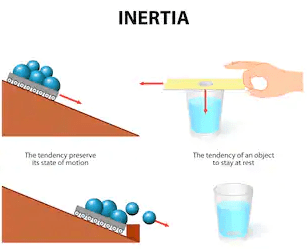Mechanics

# Newton’s First law of Motion Examples in Our Daily Lifenewton’s first law of motion examples Newton’s first law of motion states that ” A body continues its state of rest or of uniform motion in a straight line provided no net force acts on it. Newton’s 1st law of motion deals with the inertial property of matter, therefore, newton’s 1st law of motion is known as the law of inertia.
This post includes newton’s first law of motion examples.
This also includes:

• Real-life examples
• Applications
• more

So, if you want to get benefits from this post, you’ll love this.
Let’s get started…
This law consists of two parts i.e, if a body is at rest it will remain at rest until it is acted upon by an external force.
For example:
A book lying on a table or a table lying in a room, they will not change their position until an external force acts on it.
The other part of the law is “if a body is in uniform motion in a straight line it will continue its state until it is acted upon an external force.
For example:
When we roll a ball on the surface it will come to rest due to friction and air resistance. If these forces do not oppose the motion of the ball, it will continue its uniform motion. From the above discussion, we reach the following two very important concepts.

1. The definition of force i.e, it is the force that can change the position of the object or it is the force that can stop a moving ball.
2. The inertia of a body.

All material objects possess the property of opposing any change in their state of rest or uniform motion in a straight line. So, we conclude that if there is no external force acting on a body it will be in an inertial frame. In other words, inertia is the tendency of an object to resist the change in its state of rest or uniform motion in a straight line”.Therefore the first law of motion is also known as the law of inertia.

### Newton’s First Law of motion examples

Here are some examples of Newton’s first law of motion  are given in the list:

1. A driver of automobile brakes abruptly and, by inertia, shoots forward.
2. A stone in the ground is in a state of rest.
3. A bicycle stored five years ago in a loft comes out of its inertia when a child decides to use it.
4. A marathoner continues to run several meters beyond the finish line due to the inertia of his career.
5. A book lying on the table remains at rest as long as no net force acts on it.
6. A moving object does not stop moving by itself.
7. A rolling ball on a rough surface or ground stops earlier than on a smooth surface because rough surfaces offer more friction than a smooth surface. If there would no force to oppose then the motion of a body then the moving body would never stop.

### Law of inertia (explanation):A block of stone that is hardly be pushed along the ground while a small wooden block can easily be pushed along the ground. The mass of the stone or wooden block is the mass of inertia.
For example:
A person riding a bicycle along a leveled road if stops pedaling does not come to rest immediately. The bicycle continues to move forward due to inertia. A small coin is put on a card and placed over the mouth of a glass. When the card is flicked away with the finger horizontally, the coin drops neatly into the glass due to inertia.

### Why we need newton 1st law of motion?

From the section of forces, we discussed some properties of forces, but from that section, we said nothing about how forces affect motion.
Related topics: